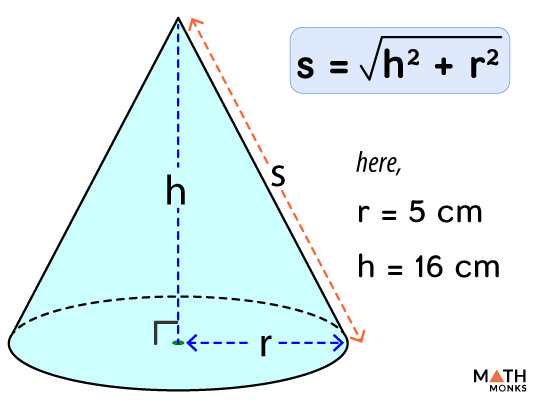# How to Find the Height of a Cone

The height of a cone is the perpendicular line connecting the vertex and the base. For a right circular cone, it is the perpendicular joining the vertex and the center of the base. It is denoted by ‘h’.

Another parameter of a cone is its slant height. It is the minimum length from the vertex to the outer edge of the base. It is denoted by ‘l’ or ‘s’.

Since height is a linear parameter, it is expressed in mm, cm, m, in, or ft.

## Formulas

As we know,

Volume ${\left( V\right) =\dfrac{1}{3}\pi r^{2}h}$, here r = radius, h = height, π = 3.141

We can derive the formula to find the height (h) by rearranging the equation as follows.

Height ${(h)=\dfrac{3V}{\pi r^{2}}}$

To find the slant height we apply the Pythagorean’s theorem. We consider height (h) as the perpendicular leg of a right triangle, the radius (r) as the base, and the slant height (s) as the hypotenuse.

The formula is:

Slant Height ${\left( s\right) =\sqrt{h^{2}+r^{2}}}$

Find the height of a cone with a volume of 975 mm3 and a radius of 7 mm.

Solution:

As we know,
Height ${h=\dfrac{3V}{\pi r^{2}}}$, here V = 975 mm3, r = 7 mm
${= \dfrac{3\times 975}{3.141\times 7^{2}}}$
= 19 mm

Finding the HEIGHT of a cone when the SLANT HEIGHT and RADIUS are known

Calculate the height of a cone with a slant height of 8 cm and a radius of 7 cm.

Solution:

Here, we will use the alternative formula using the Pythagorean’s theorem.
${\therefore h=\sqrt{s^{2}-r^{2}}}$, here s = 8 cm, r = 7 cm
${\therefore h=\sqrt{8^{2}-7^{2}}}$
= 3.87 cmFind the slant height of a cone given in the figure alongside.

Solution:

We will use the data given in the figure.
As we know,
Slant Height ${\left( s\right) =\sqrt{h^{2}+r^{2}}}$, here h = 5 cm, r = 16 cm
${\therefore s=\sqrt{5^{2}+16^{2}}}$
= 16.76 cm